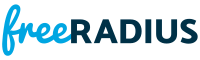heap.h File Reference

Structures and prototypes for binary heaps. More...

Go to the source code of this file.

Typedefs

typedef int(* fr_heap_cmp_t )(void const *, void const *)

typedef struct fr_heap_t fr_heap_t

Functions

fr_heap_tfr_heap_create (fr_heap_cmp_t cmp, size_t offset)

void fr_heap_delete (fr_heap_t *hp)

int fr_heap_extract (fr_heap_t *hp, void *data)

int fr_heap_insert (fr_heap_t *hp, void *data)

size_t fr_heap_num_elements (fr_heap_t *hp)

void * fr_heap_peek (fr_heap_t *hp)

Detailed Description

Structures and prototypes for binary heaps.

Id:
232bc19fbe4ac66387059f5c803b64f07bc9a31c

Definition in file heap.h.

Typedef Documentation

 typedef int(* fr_heap_cmp_t)(void const *, void const *)

Definition at line 32 of file heap.h.

 typedef struct fr_heap_t fr_heap_t

Definition at line 34 of file heap.h.

Function Documentation

 fr_heap_t* fr_heap_create ( fr_heap_cmp_t cmp, size_t offset )

Definition at line 44 of file heap.c.Here is the caller graph for this function:

 void fr_heap_delete ( fr_heap_t * hp )

Definition at line 36 of file heap.c.Here is the caller graph for this function:

 int fr_heap_extract ( fr_heap_t * hp, void * data )

Definition at line 147 of file heap.c.Here is the call graph for this function:Here is the caller graph for this function:

 int fr_heap_insert ( fr_heap_t * hp, void * data )

Definition at line 92 of file heap.c.Here is the call graph for this function:Here is the caller graph for this function:

 size_t fr_heap_num_elements ( fr_heap_t * hp )

Definition at line 217 of file heap.c.Here is the caller graph for this function:

 void* fr_heap_peek ( fr_heap_t * hp )

Definition at line 207 of file heap.c.Here is the caller graph for this function: